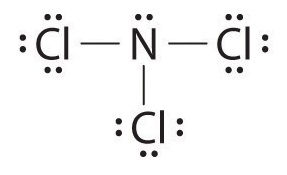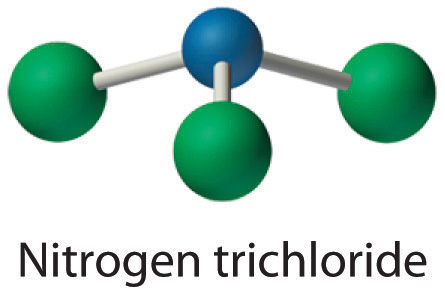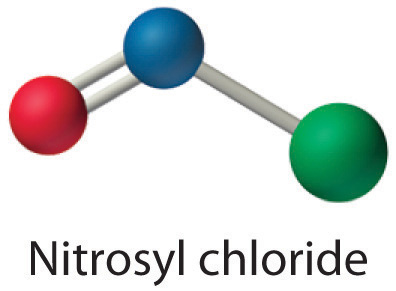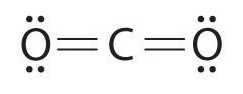# 9.4: Writing Lewis Structures

$$\newcommand{\vecs}{\overset { \rightharpoonup} {\mathbf{#1}} }$$ $$\newcommand{\vecd}{\overset{-\!-\!\rightharpoonup}{\vphantom{a}\smash {#1}}}$$$$\newcommand{\id}{\mathrm{id}}$$ $$\newcommand{\Span}{\mathrm{span}}$$ $$\newcommand{\kernel}{\mathrm{null}\,}$$ $$\newcommand{\range}{\mathrm{range}\,}$$ $$\newcommand{\RealPart}{\mathrm{Re}}$$ $$\newcommand{\ImaginaryPart}{\mathrm{Im}}$$ $$\newcommand{\Argument}{\mathrm{Arg}}$$ $$\newcommand{\norm}{\| #1 \|}$$ $$\newcommand{\inner}{\langle #1, #2 \rangle}$$ $$\newcommand{\Span}{\mathrm{span}}$$ $$\newcommand{\id}{\mathrm{id}}$$ $$\newcommand{\Span}{\mathrm{span}}$$ $$\newcommand{\kernel}{\mathrm{null}\,}$$ $$\newcommand{\range}{\mathrm{range}\,}$$ $$\newcommand{\RealPart}{\mathrm{Re}}$$ $$\newcommand{\ImaginaryPart}{\mathrm{Im}}$$ $$\newcommand{\Argument}{\mathrm{Arg}}$$ $$\newcommand{\norm}{\| #1 \|}$$ $$\newcommand{\inner}{\langle #1, #2 \rangle}$$ $$\newcommand{\Span}{\mathrm{span}}$$

Learning Objectives

• To use Lewis dot symbols to explain the stoichiometry of a compound

## Using Lewis Dot Symbols to Describe Covalent Bonding

The valence electron configurations of the constituent atoms of a covalent compound are important factors in determining its structure, stoichiometry, and properties. For example, chlorine, with seven valence electrons, is one electron short of an octet. If two chlorine atoms share their unpaired electrons by making a covalent bond and forming Cl2, they can each complete their valence shell:Each chlorine atom now has an octet. The electron pair being shared by the atoms is called a bonding pair; the other three pairs of electrons on each chlorine atom are called lone pairs. Lone pairs are not involved in covalent bonding. If both electrons in a covalent bond come from the same atom, the bond is called a coordinate covalent bond. Examples of this type of bonding are presented in Section 8.6 when we discuss atoms with less than an octet of electrons.

We can illustrate the formation of a water molecule from two hydrogen atoms and an oxygen atom using Lewis dot symbols:The structure on the right is the Lewis electron structure, or Lewis structure, for H2O. With two bonding pairs and two lone pairs, the oxygen atom has now completed its octet. Moreover, by sharing a bonding pair with oxygen, each hydrogen atom now has a full valence shell of two electrons. Chemists usually indicate a bonding pair by a single line, as shown here for our two examples:The following procedure can be used to construct Lewis electron structures for more complex molecules and ions:

1. Arrange the atoms to show specific connections. When there is a central atom, it is usually the least electronegative element in the compound. Chemists usually list this central atom first in the chemical formula (as in CCl4 and CO32, which both have C as the central atom), which is another clue to the compound’s structure. Hydrogen and the halogens are almost always connected to only one other atom, so they are usually terminal rather than central.
2. Determine the total number of valence electrons in the molecule or ion. Add together the valence electrons from each atom. (Recall that the number of valence electrons is indicated by the position of the element in the periodic table.) If the species is a polyatomic ion, remember to add or subtract the number of electrons necessary to give the total charge on the ion. For CO32, for example, we add two electrons to the total because of the −2 charge.
3. Place a bonding pair of electrons between each pair of adjacent atoms to give a single bond. In H2O, for example, there is a bonding pair of electrons between oxygen and each hydrogen.
4. Beginning with the terminal atoms, add enough electrons to each atom to give each atom an octet (two for hydrogen). These electrons will usually be lone pairs.
5. If any electrons are left over, place them on the central atom. We will explain later that some atoms are able to accommodate more than eight electrons.
6. If the central atom has fewer electrons than an octet, use lone pairs from terminal atoms to form multiple (double or triple) bonds to the central atom to achieve an octet. This will not change the number of electrons on the terminal atoms.

Now let’s apply this procedure to some particular compounds, beginning with one we have already discussed.

Note

The central atom is usually the least electronegative element in the molecule or ion; hydrogen and the halogens are usually terminal.

### The $$H_2O$$ Molecule

1. Because H atoms are almost always terminal, the arrangement within the molecule must be HOH.
2. Each H atom (group 1) has 1 valence electron, and the O atom (group 16) has 6 valence electrons, for a total of 8 valence electrons.
3. Placing one bonding pair of electrons between the O atom and each H atom gives H:O:H, with 4 electrons left over.
4. Each H atom has a full valence shell of 2 electrons.
5. Adding the remaining 4 electrons to the oxygen (as two lone pairs) gives the following structure:This is the Lewis structure we drew earlier. Because it gives oxygen an octet and each hydrogen two electrons, we do not need to use step 6.

### The $$OCl^−$$ Ion

1. With only two atoms in the molecule, there is no central atom.
2. Oxygen (group 16) has 6 valence electrons, and chlorine (group 17) has 7 valence electrons; we must add one more for the negative charge on the ion, giving a total of 14 valence electrons.
3. Placing a bonding pair of electrons between O and Cl gives O:Cl, with 12 electrons left over.
4. If we place six electrons (as three lone pairs) on each atom, we obtain the following structure:Each atom now has an octet of electrons, so steps 5 and 6 are not needed. The Lewis electron structure is drawn within brackets as is customary for an ion, with the overall charge indicated outside the brackets, and the bonding pair of electrons is indicated by a solid line. OCl is the hypochlorite ion, the active ingredient in chlorine laundry bleach and swimming pool disinfectant.

### The $$CH_2O$$ Molecule

1. Because carbon is less electronegative than oxygen and hydrogen is normally terminal, C must be the central atom. One possible arrangement is as follows:2. Each hydrogen atom (group 1) has one valence electron, carbon (group 14) has 4 valence electrons, and oxygen (group 16) has 6 valence electrons, for a total of [(2)(1) + 4 + 6] = 12 valence electrons.

3. Placing a bonding pair of electrons between each pair of bonded atoms gives the following:Six electrons are used, and 6 are left over.

4. Adding all 6 remaining electrons to oxygen (as three lone pairs) gives the following:Although oxygen now has an octet and each hydrogen has 2 electrons, carbon has only 6 electrons.

5. There are no electrons left to place on the central atom.

6. To give carbon an octet of electrons, we use one of the lone pairs of electrons on oxygen to form a carbon–oxygen double bond:Both the oxygen and the carbon now have an octet of electrons, so this is an acceptable Lewis electron structure. The O has two bonding pairs and two lone pairs, and C has four bonding pairs. This is the structure of formaldehyde, which is used in embalming fluid. An alternative structure can be drawn with one H bonded to O. Formal charges, discussed later in this section, suggest that such a structure is less stable than that shown previously.

Example $$\PageIndex{1}$$

Write the Lewis electron structure for each species.

1. NCl3
2. S22
3. NOCl

Given: chemical species

Strategy:

Use the six-step procedure to write the Lewis electron structure for each species.

Solution:

1. Nitrogen is less electronegative than chlorine, and halogen atoms are usually terminal, so nitrogen is the central atom. The nitrogen atom (group 15) has 5 valence electrons and each chlorine atom (group 17) has 7 valence electrons, for a total of 26 valence electrons. Using 2 electrons for each N–Cl bond and adding three lone pairs to each Cl account for (3 × 2) + (3 × 2 × 3) = 24 electrons. Rule 5 leads us to place the remaining 2 electrons on the central N:Nitrogen trichloride is an unstable oily liquid once used to bleach flour; this use is now prohibited in the United States.2. In a diatomic molecule or ion, we do not need to worry about a central atom. Each sulfur atom (group 16) contains 6 valence electrons, and we need to add 2 electrons for the −2 charge, giving a total of 14 valence electrons. Using 2 electrons for the S–S bond, we arrange the remaining 12 electrons as three lone pairs on each sulfur, giving each S atom an octet of electrons:3. Because nitrogen is less electronegative than oxygen or chlorine, it is the central atom. The N atom (group 15) has 5 valence electrons, the O atom (group 16) has 6 valence electrons, and the Cl atom (group 17) has 7 valence electrons, giving a total of 18 valence electrons. Placing one bonding pair of electrons between each pair of bonded atoms uses 4 electrons and gives the following:Adding three lone pairs each to oxygen and to chlorine uses 12 more electrons, leaving 2 electrons to place as a lone pair on nitrogen:Because this Lewis structure has only 6 electrons around the central nitrogen, a lone pair of electrons on a terminal atom must be used to form a bonding pair. We could use a lone pair on either O or Cl. Because we have seen many structures in which O forms a double bond but none with a double bond to Cl, it is reasonable to select a lone pair from O to give the following:All atoms now have octet configurations. This is the Lewis electron structure of nitrosyl chloride, a highly corrosive, reddish-orange gas.Exercise $$\PageIndex{1}$$

Write Lewis electron structures for CO2 and SCl2, a vile-smelling, unstable red liquid that is used in the manufacture of rubber.

1.2.Lewis Structure of Molecules: https://youtu.be/xWiFCqA9Ur0

## Using Lewis Electron Structures to Explain Stoichiometry

Lewis dot symbols provide a simple rationalization of why elements form compounds with the observed stoichiometries. In the Lewis model, the number of bonds formed by an element in a neutral compound is the same as the number of unpaired electrons it must share with other atoms to complete its octet of electrons. For the elements of Group 17 (the halogens), this number is one; for the elements of Group 16 (the chalcogens), it is two; for Group 15 elements, three; and for Group 14 elements four. These requirements are illustrated by the following Lewis structures for the hydrides of the lightest members of each group:Elements may form multiple bonds to complete an octet. In ethylene, for example, each carbon contributes two electrons to the double bond, giving each carbon an octet (two electrons/bond × four bonds = eight electrons). Neutral structures with fewer or more bonds exist, but they are unusual and violate the octet rule.Allotropes of an element can have very different physical and chemical properties because of different three-dimensional arrangements of the atoms; the number of bonds formed by the component atoms, however, is always the same. As noted at the beginning of the chapter, diamond is a hard, transparent solid; graphite is a soft, black solid; and the fullerenes have open cage structures. Despite these differences, the carbon atoms in all three allotropes form four bonds, in accordance with the octet rule.

Note

Lewis structures explain why the elements of groups 14–17 form neutral compounds with four, three, two, and one bonded atom(s), respectively.

Elemental phosphorus also exists in three forms: white phosphorus, a toxic, waxy substance that initially glows and then spontaneously ignites on contact with air; red phosphorus, an amorphous substance that is used commercially in safety matches, fireworks, and smoke bombs; and black phosphorus, an unreactive crystalline solid with a texture similar to graphite (Figure $$\PageIndex{3}$$). Nonetheless, the phosphorus atoms in all three forms obey the octet rule and form three bonds per phosphorus atom.Figure $$\PageIndex{3}$$: The Three Allotropes of Phosphorus: White, Red, and Black. ll three forms contain only phosphorus atoms, but they differ in the arrangement and connectivity of their atoms. White phosphorus contains P4 tetrahedra, red phosphorus is a network of linked P8 and P9 units, and black phosphorus forms sheets of six-membered rings. As a result, their physical and chemical properties differ dramatically.

## Formal Charges

It is sometimes possible to write more than one Lewis structure for a substance that does not violate the octet rule, as we saw for CH2O, but not every Lewis structure may be equally reasonable. In these situations, we can choose the most stable Lewis structure by considering the formal charge on the atoms, which is the difference between the number of valence electrons in the free atom and the number assigned to it in the Lewis electron structure. The formal charge is a way of computing the charge distribution within a Lewis structure; the sum of the formal charges on the atoms within a molecule or an ion must equal the overall charge on the molecule or ion. A formal charge does not represent a true charge on an atom in a covalent bond but is simply used to predict the most likely structure when a compound has more than one valid Lewis structure.

To calculate formal charges, we assign electrons in the molecule to individual atoms according to these rules:

• Nonbonding electrons are assigned to the atom on which they are located.
• Bonding electrons are divided equally between the bonded atoms.

For each atom, we then compute a formal charge:

$$\begin{matrix} formal\; charge= & valence\; e^{-}- & \left ( non-bonding\; e^{-}+\frac{bonding\;e^{-}}{2} \right )\\ & ^{\left ( free\; atom \right )} & ^{\left ( atom\; in\; Lewis\; structure \right )} \end{matrix} \label{8.5.1}$$ (atom in Lewis structure)

To illustrate this method, let’s calculate the formal charge on the atoms in ammonia (NH3) whose Lewis electron structure is as follows:A neutral nitrogen atom has five valence electrons (it is in group 15). From its Lewis electron structure, the nitrogen atom in ammonia has one lone pair and shares three bonding pairs with hydrogen atoms, so nitrogen itself is assigned a total of five electrons [2 nonbonding e + (6 bonding e/2)]. Substituting into Equation 8.5.2, we obtain

$formal\; charge\left ( N \right )=5\; valence\; e^{-}-\left ( 2\; non-bonding\; e^{-} +\frac{6\; bonding\; e^{-}}{2} \right )=0 \label{8.5.2}$

A neutral hydrogen atom has one valence electron. Each hydrogen atom in the molecule shares one pair of bonding electrons and is therefore assigned one electron [0 nonbonding e + (2 bonding e/2)]. Using Equation 8.5.2 to calculate the formal charge on hydrogen, we obtain

$formal\; charge\left ( H \right )=1\; valence\; e^{-}-\left ( 0\; non-bonding\; e^{-} +\frac{2\; bonding\; e^{-}}{2} \right )=0 \label{8.5.3}$

The hydrogen atoms in ammonia have the same number of electrons as neutral hydrogen atoms, and so their formal charge is also zero. Adding together the formal charges should give us the overall charge on the molecule or ion. In this example, the nitrogen and each hydrogen has a formal charge of zero. When summed the overall charge is zero, which is consistent with the overall charge on the NH3 molecule.

Note

An atom, molecule, or ion has a formal charge of zero if it has the number of bonds that is typical for that species.

Typically, the structure with the most charges on the atoms closest to zero is the more stable Lewis structure. In cases where there are positive or negative formal charges on various atoms, stable structures generally have negative formal charges on the more electronegative atoms and positive formal charges on the less electronegative atoms. The next example further demonstrates how to calculate formal charges.

Lewis Structure of Charged Molecules: https://youtu.be/pTkziPtvMYU

Example $$\PageIndex{2}$$

Calculate the formal charges on each atom in the NH4+ ion.

Given: chemical species

Strategy:

Identify the number of valence electrons in each atom in the NH4+ ion. Use the Lewis electron structure of NH4+ to identify the number of bonding and nonbonding electrons associated with each atom and then use Equation 8.5.2 to calculate the formal charge on each atom.

Solution:

The Lewis electron structure for the NH4+ ion is as follows:The nitrogen atom shares four bonding pairs of electrons, and a neutral nitrogen atom has five valence electrons. Using Equation 8.5.1, the formal charge on the nitrogen atom is therefore

$formal\; charge\left ( N \right )=5-\left ( 0+\frac{8}{2} \right )=0$

Each hydrogen atom in has one bonding pair. The formal charge on each hydrogen atom is therefore

$formal\; charge\left ( H \right )=1-\left ( 0+\frac{2}{2} \right )=0$

The formal charges on the atoms in the NH4+ ion are thusAdding together the formal charges on the atoms should give us the total charge on the molecule or ion. In this case, the sum of the formal charges is 0 + 1 + 0 + 0 + 0 = +1.

Exercise $$\PageIndex{2}$$

Write the formal charges on all atoms in BH4.If an atom in a molecule or ion has the number of bonds that is typical for that atom (e.g., four bonds for carbon), its formal charge is zero.

## Using Formal Charges to Distinguish Viable Lewis Structures

As an example of how formal charges can be used to determine the most stable Lewis structure for a substance, we can compare two possible structures for CO2. Both structures conform to the rules for Lewis electron structures.

### CO2

1. C is less electronegative than O, so it is the central atom.
2. C has 4 valence electrons and each O has 6 valence electrons, for a total of 16 valence electrons.
3. Placing one electron pair between the C and each O gives O–C–O, with 12 electrons left over.
4. Dividing the remaining electrons between the O atoms gives three lone pairs on each atom:This structure has an octet of electrons around each O atom but only 4 electrons around the C atom.

1. No electrons are left for the central atom.
2. To give the carbon atom an octet of electrons, we can convert two of the lone pairs on the oxygen atoms to bonding electron pairs. There are, however, two ways to do this. We can either take one electron pair from each oxygen to form a symmetrical structure or take both electron pairs from a single oxygen atom to give an asymmetrical structure:Both Lewis electron structures give all three atoms an octet. How do we decide between these two possibilities? The formal charges for the two Lewis electron structures of CO2 are as follows:Both Lewis structures have a net formal charge of zero, but the structure on the right has a +1 charge on the more electronegative atom (O). Thus the symmetrical Lewis structure on the left is predicted to be more stable, and it is, in fact, the structure observed experimentally. Remember, though, that formal charges do not represent the actual charges on atoms in a molecule or ion. They are used simply as a bookkeeping method for predicting the most stable Lewis structure for a compound.

Note

The Lewis structure with the set of formal charges closest to zero is usually the most stable.

Example $$\PageIndex{3}$$: The Thiocyanate Ion

The thiocyanate ion (SCN), which is used in printing and as a corrosion inhibitor against acidic gases, has at least two possible Lewis electron structures. Draw two possible structures, assign formal charges on all atoms in both, and decide which is the preferred arrangement of electrons.

Given: chemical species

Asked for: Lewis electron structures, formal charges, and preferred arrangement

Strategy:

1. Use the step-by-step procedure to write two plausible Lewis electron structures for SCN.
2. Calculate the formal charge on each atom using Equation 8.5.1.
3. Predict which structure is preferred based on the formal charge on each atom and its electronegativity relative to the other atoms present.

Solution:

A Possible Lewis structures for the SCN ion are as follows:B We must calculate the formal charges on each atom to identify the more stable structure. If we begin with carbon, we notice that the carbon atom in each of these structures shares four bonding pairs, the number of bonds typical for carbon, so it has a formal charge of zero. Continuing with sulfur, we observe that in (a) the sulfur atom shares one bonding pair and has three lone pairs and has a total of six valence electrons. The formal charge on the sulfur atom is therefore $$6-\left ( 6+\frac{2}{2} \right )=-1.5-\left ( 4+\frac{4}{2} \right )=-1$$ In (c), nitrogen has a formal charge of −2.

C Which structure is preferred? Structure (b) is preferred because the negative charge is on the more electronegative atom (N), and it has lower formal charges on each atom as compared to structure (c): 0, −1 versus +1, −2.

Exercise $$\PageIndex{3}$$: The Fulminate Ion

Salts containing the fulminate ion (CNO) are used in explosive detonators. Draw three Lewis electron structures for CNO and use formal charges to predict which is more stable. (Note: N is the central atom.)The second structure is predicted to be more stable.

## Summary

• Lewis dot symbols provide a simple rationalization of why elements form compounds with the observed stoichiometries.

A plot of the overall energy of a covalent bond as a function of internuclear distance is identical to a plot of an ionic pair because both result from attractive and repulsive forces between charged entities. In Lewis electron structures, we encounter bonding pairs, which are shared by two atoms, and lone pairs, which are not shared between atoms. If both electrons in a covalent bond come from the same atom, the bond is called a coordinate covalent bond. Lewis structures are an attempt to rationalize why certain stoichiometries are commonly observed for the elements of particular families. Neutral compounds of group 14 elements typically contain four bonds around each atom (a double bond counts as two, a triple bond as three), whereas neutral compounds of group 15 elements typically contain three bonds. In cases where it is possible to write more than one Lewis electron structure with octets around all the nonhydrogen atoms of a compound, the formal charge on each atom in alternative structures must be considered to decide which of the valid structures can be excluded and which is the most reasonable. The formal charge is the difference between the number of valence electrons of the free atom and the number of electrons assigned to it in the compound, where bonding electrons are divided equally between the bonded atoms. The Lewis structure with the lowest formal charges on the atoms is almost always the most stable one.